Next: Other applications Up: Applications of ICA Previous: Feature extraction

### Blind deconvolution

A less direct application of the ICA methods can be found in blind deconvolution (see Section 2). Due to the fact that the values of the original signal s(t) are independent for different t, this problem can be solved using essentially the same formalism as used in ICA, as noted above. Indeed this problem can also be represented (though only approximately) by Eq. (11); then the realizations of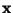and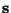are vectors containing n=m subsequent observations of the signals x(t) and s(t), beginning at different points of time. In other words, a sequence of observations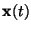is such that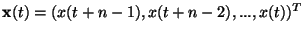for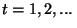. The square matrix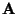is determined by the convolving filter. Though this formulation is only approximative, the exact formulation using linear filters would lead to essentially the same algorithms and convergence proofs. Also blind separation of several convolved signals ('multi-channel deconvolution') can be represented combining these two approaches, see, for example, [41,123,137,149,150,134,92].

Aapo Hyvarinen
1999-04-23